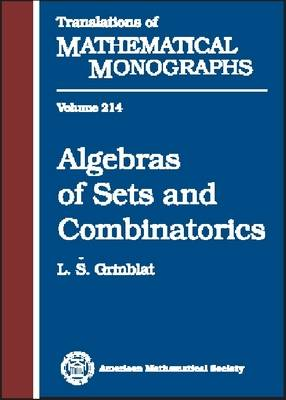•# Algebras of Sets and Combinatorics - Translations of Mathematical Monographs (Hardback)

(author)
£112.00
Hardback 264 Pages / Published: 30/09/2002
• We can order this from the publisher

UK delivery within 3-4 weeks

An algebra \$A\$ on a set \$X\$ is a family of subsets of this set closed under the operations of union and difference of two subsets. The main topic of the book is the study of various algebras and families of algebras on an abstract set \$X\$. The author shows how this is related to famous problems by Lebesgue, Banach, and Ulam on the existence of certain measures on abstract sets, with corresponding algebras being algebras of measurable subsets with respect to these measures. In particular it is shown that for a certain algebra not to coincide with the algebra of all subsets of \$X\$ is equivalent to the existence of a nonmeasurable set with respect to a given measure.Although these questions don't seem to be related to mathematical logic, many results in this area were proved by 'metamathematical' methods, using the method of forcing and other tools related to axiomatic set theory. However, in the present book, the author uses 'elementary' (mainly combinatorial) methods to study properties of algebras on a set. Presenting new and original material, the book is written in a clear and readable style and illustrated by many examples and figures. The book will be useful to researchers and graduate students working in set theory, mathematical logic, and combinatorics.

Publisher: American Mathematical Society
ISBN: 9780821827659
Number of pages: 264
Weight: 680 g